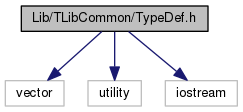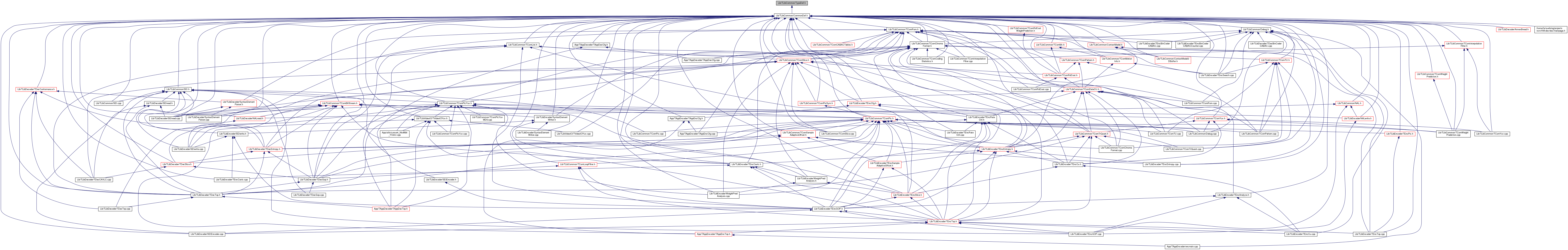HEVC Test Model (HM)  HM-16.18
TypeDef.h File Reference

Define macros, basic types, new types and enumerations. More...

`#include <vector>`
`#include <utility>`
`#include <iostream>`
Include dependency graph for TypeDef.h:This graph shows which files directly or indirectly include this file:Go to the source code of this file.

## Classes

struct  SAOOffset

struct  SAOBlkParam

struct  BitDepths

struct  _LFCUParam
parameters for deblocking filter More...

struct  TUEntropyCodingParameters

struct  TComPictureHash

struct  TComSEITimeSet

struct  TComSEIMasteringDisplay

struct  LumaLevelToDeltaQPMapping

struct  WCGChromaQPControl

class  Window

class  RNSEIWindow

Profile

Level

## Enumerations

enum  Profile::Name {
Profile::NONE = 0, Profile::MAIN = 1, Profile::MAIN10 = 2, Profile::MAINSTILLPICTURE = 3,
Profile::MAINREXT = 4, Profile::HIGHTHROUGHPUTREXT = 5
}

enum  Level::Tier { Level::MAIN = 0, Level::HIGH = 1, Level::NUMBER_OF_TIERS = 2 }

enum  Level::Name {
Level::NONE = 0, Level::LEVEL1 = 30, Level::LEVEL2 = 60, Level::LEVEL2_1 = 63,
Level::LEVEL3 = 90, Level::LEVEL3_1 = 93, Level::LEVEL4 = 120, Level::LEVEL4_1 = 123,
Level::LEVEL5 = 150, Level::LEVEL5_1 = 153, Level::LEVEL5_2 = 156, Level::LEVEL6 = 180,
Level::LEVEL6_1 = 183, Level::LEVEL6_2 = 186, Level::LEVEL8_5 = 255
}

#define DEBUG_STRING   0
When enabled, prints out final decision debug info at encoder and decoder. More...

#define DEBUG_ENCODER_SEARCH_BINS   0
When enabled, prints out each bin as it is coded during encoder search. More...

#define DEBUG_CABAC_BINS   0
When enabled, prints out each bin as it is coded during final encode and decode. More...

#define DEBUG_INTRA_SEARCH_COSTS   0
When enabled, prints out the cost for each mode during encoder search. More...

#define DEBUG_TRANSFORM_AND_QUANTISE   0
When enabled, prints out each TU as it passes through the transform-quantise-dequantise-inverseTransform process. More...

#define ENVIRONMENT_VARIABLE_DEBUG_AND_TEST   0
When enabled, allows control of debug modifications via environment variables. More...

#define PRINT_MACRO_VALUES   1
When enabled, the encoder prints out a list of the non-environment-variable controlled macros and their values on startup. More...

#define RExt__DECODER_DEBUG_BIT_STATISTICS   0
0 (default) = decoder reports as normal, 1 = decoder produces bit usage statistics (will impact decoder run time by up to ~10%) More...

#define ENC_DEC_TRACE   0

#define DEC_NUH_TRACE   0
When trace enabled, enable tracing of NAL unit headers at the decoder (currently not possible at the encoder) More...

#define PRINT_RPS_INFO   0
Enable/disable the printing of bits used to send the RPS. More...

#define DECODER_CHECK_SUBSTREAM_AND_SLICE_TRAILING_BYTES   1
TODO: integrate this macro into a broader conformance checking system. More...

#define X0038_LAMBDA_FROM_QP_CAPABILITY   1
This approach derives lambda from QP+QPoffset+QPoffset2. QPoffset2 is derived from QP+QPoffset using a linear model that is clipped between 0 and 3. More...

#define JCTVC_Y0038_PARAMS   1

#define JVET_E0059_FLOATING_POINT_QP_FIX   1
Replace floating point QP with a source-file frame number. More...

#define JVET_G0101_QP_SWITCHING   1
After switching POC, increase base QP instead of frame level QP. More...

#define JVET_F0064_MSSSIM   1
Calculate MS-SSIM scores. More...

#define EXTENSION_360_VIDEO   0
extension for 360/spherical video coding support; this macro should be controlled by makefile, as it would be used to control whether the library is built and linked More...

#define MCTS_ENC_CHECK   1
Temporal MCTS encoder constraint and decoder checks. Also requires SEITMCTSTileConstraint to be enabled to enforce constraint. More...

#define CCV_SEI_MESSAGE   1

#define RWP_SEI_MESSAGE   1

#define CMP_SEI_MESSAGE   1

#define ERP_SR_OV_SEI_MESSAGE   1

#define RNSEI   1
Support for signalling regional nesting SEI message. More...

#define REDUCED_ENCODER_MEMORY   1
When 1, the encoder will allocate TComPic memory when required and release it when no longer required. More...

#define AMP_ENC_SPEEDUP   1
encoder only speed-up by AMP mode skipping More...

#define AMP_MRG   1
encoder only force merge for AMP partition (no motion search for AMP) More...

#define FAST_BIT_EST   1
G763: Table-based bit estimation for CABAC. More...

#define HHI_RQT_INTRA_SPEEDUP   1
tests one best mode with full rqt More...

#define HHI_RQT_INTRA_SPEEDUP_MOD   0
tests two best modes with full rqt More...

#define MATRIX_MULT   0
Brute force matrix multiplication instead of partial butterfly. More...

#define O0043_BEST_EFFORT_DECODING   0
0 (default) = disable code related to best effort decoding, 1 = enable code relating to best effort decoding [ decode-side only ]. More...

#define ME_ENABLE_ROUNDING_OF_MVS   1
0 (default) = disables rounding of motion vectors when right shifted, 1 = enables rounding More...

#define RDOQ_CHROMA_LAMBDA   1
F386: weighting of chroma for RDOQ. More...

#define RExt__HIGH_BIT_DEPTH_SUPPORT   0
0 (default) use data type definitions for 8-10 bit video, 1 = use larger data types to allow for up to 16-bit video (originally developed as part of N0188) More...

#define VECTOR_CODING__INTERPOLATION_FILTER   0
enable vector coding for the interpolation filter. 0 (default if SSE not possible) disable SSE vector coding. Should not affect RD costs/decisions. Code back-ported from JEM2.0. More...

#define VECTOR_CODING__DISTORTION_CALCULATIONS   0
enable vector coding for distortion calculations 0 (default if SSE not possible) disable SSE vector coding. Should not affect RD costs/decisions. Code back-ported from JEM2.0. More...

#define FULL_NBIT   0
When enabled, use distortion measure derived from all bits of source data, otherwise discard (bitDepth - 8) least-significant bits of distortion. More...

#define RExt__HIGH_PRECISION_FORWARD_TRANSFORM   0
0 (default) use original 6-bit transform matrices for both forward and inverse transform, 1 = use original matrices for inverse transform and high precision matrices for forward transform More...

#define DEBUG_STRING_PASS_INTO(name)

#define DEBUG_STRING_PASS_INTO_OPTIONAL(name, exp)

#define DEBUG_STRING_FN_DECLARE(name)

#define DEBUG_STRING_FN_DECLAREP(name)

#define DEBUG_STRING_NEW(name)

#define DEBUG_STRING_OUTPUT(os, name)

#define DEBUG_STRING_APPEND(str1, str2)

#define DEBUG_STRING_SWAP(srt1, str2)

#define DEBUG_STRING_CHANNEL_CONDITION(compID)

#define NUM_SAO_EO_TYPES_LOG2   2

#define NUM_SAO_BO_CLASSES_LOG2   5

#define NUM_SAO_BO_CLASSES   (1<<NUM_SAO_BO_CLASSES_LOG2)

#define MAX_NUM_SAO_CLASSES   32

enum  RDPCMMode { RDPCM_OFF = 0, RDPCM_HOR = 1, RDPCM_VER = 2, NUMBER_OF_RDPCM_MODES = 3 }

enum  RDPCMSignallingMode { RDPCM_SIGNAL_IMPLICIT = 0, RDPCM_SIGNAL_EXPLICIT = 1, NUMBER_OF_RDPCM_SIGNALLING_MODES = 2 }

enum  SliceType { B_SLICE = 0, P_SLICE = 1, I_SLICE = 2, NUMBER_OF_SLICE_TYPES = 3 }
supported slice type More...

enum  ChromaFormat {
CHROMA_400 = 0, CHROMA_420 = 1, CHROMA_422 = 2, CHROMA_444 = 3,
NUM_CHROMA_FORMAT = 4
}
chroma formats (according to semantics of chroma_format_idc) More...

enum  ChannelType { CHANNEL_TYPE_LUMA = 0, CHANNEL_TYPE_CHROMA = 1, MAX_NUM_CHANNEL_TYPE = 2 }

enum  ComponentID { COMPONENT_Y = 0, COMPONENT_Cb = 1, COMPONENT_Cr = 2, MAX_NUM_COMPONENT = 3 }

enum  InputColourSpaceConversion {
IPCOLOURSPACE_UNCHANGED = 0, IPCOLOURSPACE_YCbCrtoYCrCb = 1, IPCOLOURSPACE_YCbCrtoYYY = 2, IPCOLOURSPACE_RGBtoGBR = 3,
NUMBER_INPUT_COLOUR_SPACE_CONVERSIONS = 4
}

enum  MATRIX_COEFFICIENTS {
MATRIX_COEFFICIENTS_RGB = 0, MATRIX_COEFFICIENTS_BT709 = 1, MATRIX_COEFFICIENTS_UNSPECIFIED = 2, MATRIX_COEFFICIENTS_RESERVED_BY_ITUISOIEC = 3,
MATRIX_COEFFICIENTS_USFCCT47 = 4, MATRIX_COEFFICIENTS_BT601_625 = 5, MATRIX_COEFFICIENTS_BT601_525 = 6, MATRIX_COEFFICIENTS_SMPTE240 = 7,
MATRIX_COEFFICIENTS_YCGCO = 8, MATRIX_COEFFICIENTS_BT2020_NON_CONSTANT_LUMINANCE = 9, MATRIX_COEFFICIENTS_BT2020_CONSTANT_LUMINANCE = 10
}

enum  DeblockEdgeDir { EDGE_VER = 0, EDGE_HOR = 1, NUM_EDGE_DIR = 2 }

enum  PartSize {
SIZE_2Nx2N = 0, SIZE_2NxN = 1, SIZE_Nx2N = 2, SIZE_NxN = 3,
SIZE_2NxnU = 4, SIZE_2NxnD = 5, SIZE_nLx2N = 6, SIZE_nRx2N = 7,
NUMBER_OF_PART_SIZES = 8
}
supported partition shape More...

enum  PredMode { MODE_INTER = 0, MODE_INTRA = 1, NUMBER_OF_PREDICTION_MODES = 2 }
supported prediction type More...

enum  RefPicList { REF_PIC_LIST_0 = 0, REF_PIC_LIST_1 = 1, NUM_REF_PIC_LIST_01 = 2, REF_PIC_LIST_X = 100 }
reference list index More...

enum  DFunc {
DF_DEFAULT = 0, DF_SSE = 1, DF_SSE4 = 2, DF_SSE8 = 3,
DF_SSE16 = 4, DF_SSE32 = 5, DF_SSE64 = 6, DF_SSE16N = 7,
DF_TOTAL_FUNCTIONS = 64
}
distortion function index More...

enum  CI_IDX {
CI_CURR_BEST = 0, CI_NEXT_BEST, CI_TEMP_BEST, CI_CHROMA_INTRA,
CI_QT_TRAFO_TEST, CI_QT_TRAFO_ROOT, CI_NUM
}
index for SBAC based RD optimization More...

enum  MVP_DIR {
MD_LEFT = 0, MD_ABOVE, MD_ABOVE_RIGHT, MD_BELOW_LEFT,
MD_ABOVE_LEFT
}
motion vector predictor direction used in AMVP More...

enum  StoredResidualType { RESIDUAL_RECONSTRUCTED = 0, RESIDUAL_ENCODER_SIDE = 1, NUMBER_OF_STORED_RESIDUAL_TYPES = 2 }

enum  TransformDirection { TRANSFORM_FORWARD = 0, TRANSFORM_INVERSE = 1, TRANSFORM_NUMBER_OF_DIRECTIONS = 2 }

enum  MESearchMethod {
MESEARCH_FULL = 0, MESEARCH_DIAMOND = 1, MESEARCH_SELECTIVE = 2, MESEARCH_DIAMOND_ENHANCED = 3,
MESEARCH_NUMBER_OF_METHODS = 4
}
supported ME search methods More...

enum  COEFF_SCAN_TYPE { SCAN_DIAG = 0, SCAN_HOR = 1, SCAN_VER = 2, SCAN_NUMBER_OF_TYPES = 3 }
coefficient scanning type used in ACS More...

enum  COEFF_SCAN_GROUP_TYPE { SCAN_UNGROUPED = 0, SCAN_GROUPED_4x4 = 1, SCAN_NUMBER_OF_GROUP_TYPES = 2 }

enum  SignificanceMapContextType {
CONTEXT_TYPE_4x4 = 0, CONTEXT_TYPE_8x8 = 1, CONTEXT_TYPE_NxN = 2, CONTEXT_TYPE_SINGLE = 3,
CONTEXT_NUMBER_OF_TYPES = 4
}

enum  ScalingListMode { SCALING_LIST_OFF, SCALING_LIST_DEFAULT, SCALING_LIST_FILE_READ }

enum  ScalingListSize {
SCALING_LIST_4x4 = 0, SCALING_LIST_8x8, SCALING_LIST_16x16, SCALING_LIST_32x32,
SCALING_LIST_SIZE_NUM
}

enum  SliceConstraint {
NO_SLICES = 0, FIXED_NUMBER_OF_CTU = 1, FIXED_NUMBER_OF_BYTES = 2, FIXED_NUMBER_OF_TILES = 3,
NUMBER_OF_SLICE_CONSTRAINT_MODES = 4
}

enum  HashType {
HASHTYPE_MD5 = 0, HASHTYPE_CRC = 1, HASHTYPE_CHECKSUM = 2, HASHTYPE_NONE = 3,
NUMBER_OF_HASHTYPES = 4
}

enum  SAOMode { SAO_MODE_OFF = 0, SAO_MODE_NEW, SAO_MODE_MERGE, NUM_SAO_MODES }

enum  SAOModeMergeTypes { SAO_MERGE_LEFT =0, SAO_MERGE_ABOVE, NUM_SAO_MERGE_TYPES }

enum  SAOModeNewTypes {
SAO_TYPE_START_EO =0, SAO_TYPE_EO_0 = SAO_TYPE_START_EO, SAO_TYPE_EO_90, SAO_TYPE_EO_135,
SAO_TYPE_EO_45, SAO_TYPE_START_BO, SAO_TYPE_BO = SAO_TYPE_START_BO, NUM_SAO_NEW_TYPES
}

enum  SAOEOClasses {
SAO_CLASS_EO_FULL_VALLEY = 0, SAO_CLASS_EO_HALF_VALLEY = 1, SAO_CLASS_EO_PLAIN = 2, SAO_CLASS_EO_HALF_PEAK = 3,
SAO_CLASS_EO_FULL_PEAK = 4, NUM_SAO_EO_CLASSES
}

enum  CostMode { COST_STANDARD_LOSSY = 0, COST_SEQUENCE_LEVEL_LOSSLESS = 1, COST_LOSSLESS_CODING = 2, COST_MIXED_LOSSLESS_LOSSY_CODING = 3 }

enum  WeightedPredictionMethod {
WP_PER_PICTURE_WITH_SIMPLE_DC_COMBINED_COMPONENT =0, WP_PER_PICTURE_WITH_SIMPLE_DC_PER_COMPONENT =1, WP_PER_PICTURE_WITH_HISTOGRAM_AND_PER_COMPONENT =2, WP_PER_PICTURE_WITH_HISTOGRAM_AND_PER_COMPONENT_AND_CLIPPING =3,
WP_PER_PICTURE_WITH_HISTOGRAM_AND_PER_COMPONENT_AND_CLIPPING_AND_EXTENSION =4
}

enum  FastInterSearchMode { FASTINTERSEARCH_DISABLED = 0, FASTINTERSEARCH_MODE1 = 1, FASTINTERSEARCH_MODE2 = 2, FASTINTERSEARCH_MODE3 = 3 }

enum  SPSExtensionFlagIndex { SPS_EXT__REXT = 0, NUM_SPS_EXTENSION_FLAGS = 8 }

enum  PPSExtensionFlagIndex { PPS_EXT__REXT = 0, NUM_PPS_EXTENSION_FLAGS = 8 }

enum  NalUnitType {
NAL_UNIT_CODED_SLICE_TRAIL_N = 0, NAL_UNIT_CODED_SLICE_TRAIL_R, NAL_UNIT_CODED_SLICE_TSA_N, NAL_UNIT_CODED_SLICE_TSA_R,
NAL_UNIT_CODED_SLICE_RASL_N, NAL_UNIT_CODED_SLICE_RASL_R, NAL_UNIT_RESERVED_VCL_N10, NAL_UNIT_RESERVED_VCL_R11,
NAL_UNIT_RESERVED_VCL_N12, NAL_UNIT_RESERVED_VCL_R13, NAL_UNIT_RESERVED_VCL_N14, NAL_UNIT_RESERVED_VCL_R15,
NAL_UNIT_CODED_SLICE_IDR_N_LP, NAL_UNIT_CODED_SLICE_CRA, NAL_UNIT_RESERVED_IRAP_VCL22, NAL_UNIT_RESERVED_IRAP_VCL23,
NAL_UNIT_RESERVED_VCL24, NAL_UNIT_RESERVED_VCL25, NAL_UNIT_RESERVED_VCL26, NAL_UNIT_RESERVED_VCL27,
NAL_UNIT_RESERVED_VCL28, NAL_UNIT_RESERVED_VCL29, NAL_UNIT_RESERVED_VCL30, NAL_UNIT_RESERVED_VCL31,
NAL_UNIT_VPS, NAL_UNIT_SPS, NAL_UNIT_PPS, NAL_UNIT_ACCESS_UNIT_DELIMITER,
NAL_UNIT_EOS, NAL_UNIT_EOB, NAL_UNIT_FILLER_DATA, NAL_UNIT_PREFIX_SEI,
NAL_UNIT_SUFFIX_SEI, NAL_UNIT_RESERVED_NVCL41, NAL_UNIT_RESERVED_NVCL42, NAL_UNIT_RESERVED_NVCL43,
NAL_UNIT_RESERVED_NVCL44, NAL_UNIT_RESERVED_NVCL45, NAL_UNIT_RESERVED_NVCL46, NAL_UNIT_RESERVED_NVCL47,
NAL_UNIT_UNSPECIFIED_48, NAL_UNIT_UNSPECIFIED_49, NAL_UNIT_UNSPECIFIED_50, NAL_UNIT_UNSPECIFIED_51,
NAL_UNIT_UNSPECIFIED_52, NAL_UNIT_UNSPECIFIED_53, NAL_UNIT_UNSPECIFIED_54, NAL_UNIT_UNSPECIFIED_55,
NAL_UNIT_UNSPECIFIED_56, NAL_UNIT_UNSPECIFIED_57, NAL_UNIT_UNSPECIFIED_58, NAL_UNIT_UNSPECIFIED_59,
NAL_UNIT_UNSPECIFIED_60, NAL_UNIT_UNSPECIFIED_61, NAL_UNIT_UNSPECIFIED_62, NAL_UNIT_UNSPECIFIED_63,
NAL_UNIT_INVALID
}

enum  LumaLevelToDQPMode { LUMALVL_TO_DQP_DISABLED = 0, LUMALVL_TO_DQP_AVG_METHOD = 1, LUMALVL_TO_DQP_MAX_METHOD = 2, LUMALVL_TO_DQP_NUM_MODES = 3 }

typedef void Void

typedef bool Bool

typedef char TChar

typedef signed char SChar

typedef unsigned char UChar

typedef short Short

typedef unsigned short UShort

typedef int Int

typedef unsigned int UInt

typedef double Double

typedef float Float

typedef long long Int64

typedef unsigned long long UInt64

typedef Short Pel
pixel type More...

typedef Int TCoeff
transform coefficient More...

typedef Short TMatrixCoeff
transform matrix coefficient More...

typedef Short TFilterCoeff
filter coefficient More...

typedef Int Intermediate_Int
used as intermediate value in calculations More...

typedef UInt Intermediate_UInt
used as intermediate value in calculations More...

typedef UInt Distortion
distortion measurement More...

typedef struct _LFCUParam LFCUParam
parameters for deblocking filter More...

typedef std::vector< RNSEIWindowRNSEIWindowVec

## Detailed Description

Define macros, basic types, new types and enumerations.

Definition in file TypeDef.h.